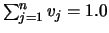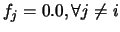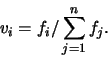Next: Manual Correction Up: Construction Previous: Preprocessing

### Classification

The goal of the classification step was to correctly label as many brain voxels as possible to minimize later manual intervention. A total of four thousand training points were selected by a trained neuroanatomist (NJK) for the following classes: 1) grey-matter (GMc), 2) white-matter (WMc), 3) cerebro-spinal fluid (CSFc), 4) fat (FATc) and 5) background (BKGc). (The c'' differentiates volumes output from the classifier from those finally used to build the phantom after manual correction.) These training points were used to drive 4 different automatic classification procedures : minimum distance, nearest K-mean, fuzzy C-mean  and ANN (artificial neural net) . The minimum distance (also known as nearest mean) and ANN classifications were selected by the neuroanatomist as the best in that they yielded the best representation for basal ganglia, cortical grey matter and white matter within the cerebellum and they required the least number of corrections. Since these two classifiers gave essentially equivalent results due to the high SNR of the images which resulted in good class separability, we chose to base the creation of the phantom on the result of the simpler minimum distance classifier.

Classification of CJH27 yielded the spatial distribution of different tissue types necessary to realistically represent the human brain. However, a discrete classification, with only one tissue label per voxel, did not allow PVEs to be modelled. In order to account for tissue mixing, it was necessary to compute and represent fractional amounts for each tissue type within each voxel. The latter was accomplished by using a volume with n-dimensional vector-valued voxels, where n was the number of tissue types represented in the phantom. The ith component of a voxel, vi, represents the fraction of ith tissue type within the voxel with the constraint 0.0 <= vi <= 1.0 and that |v| was normalized, i.e.. Practically, the phantom was represented by ten volumes, each one representing the amount of tissue i (e.g, grey matter, white matter, CSF, muscle, etc...) found at each voxel location.

We changed the standard minimum distance classifier to have a continous output. Our fuzzy'' minimum distance classifier yields tissue fractions for each voxel based on the distances computed by the classifier instead of giving only the standard discrete label output. If the voxel's intensity, g, was equal to any class mean, mi, then the ith tissue fraction, fi, was set to 1.0 and all other tissue fractions were set to 0.0 (). Otherwise, fi for each class i was estimated to be inversely proportional to the distance between the voxel's intensity and the class mean:

 fi = 1 / | g - mi |. (1)

Second, these values were normalized and stored in the vector v:(2)

This implied that all classes had some non-zero representation within each voxel. User intervention and a priori anatomical knowledge were used to zero tissue probabilities for voxels where certain tissues are known not to exist and to reassign incorrectly labelled voxels. Figure 2 shows the continous output of the fuzzy minimum distance classifier before any corrections were made.Next: Manual Correction Up: Construction Previous: Preprocessing
Louis COLLINS
1998-07-21